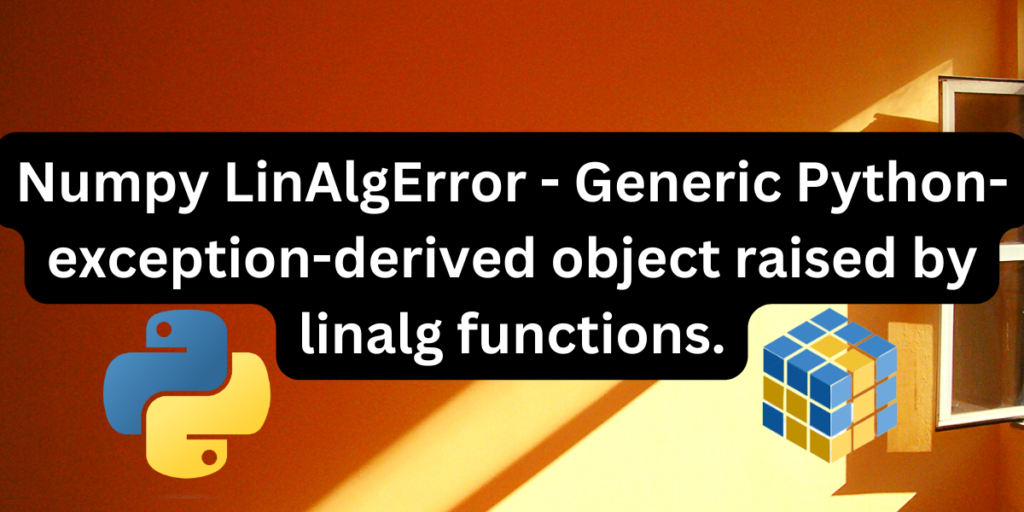# Numpy LinAlgError – Handling Matrix-related ErrorsIn python, the Base exception is the class from which all instances of exceptions are derived. Exceptions may be raised by built-in functions or the interpreter or both. User-defined programs can also raise exceptions . One such example of a built-in exception is the LinAlgError. The LinAlgError is raised by the linalg or the linear algebraic functions which mainly involve matrices.

It is a generic-python-exception- derived object raised by the linalg functions. This exception class is raised when a linear algebraic condition hinders the correct execution of a specific linalg function.

## Understanding Singular Matrices and the ‘Singular Matrix Error’ in Python

This exception is mainly raised when a matrix is singular but an in-built function or user defined block of code tries to invert it. Hence, when the determinant of a matrix is zero and you try to invert it, python will raise this exception .

A singular matrix is often referred to as a degenerate matrix because it is invertible in nature. If the determinant of a matrix is non-zero, the matrix is non-singular in nature and can be inverted.

A matrix that is positive definite can be inverted.

## How does the Error look like?

For example, let’s say you have matrix as follows:

```A=[[1,2],[-2,-4]]
```

Now, if you try to invert this matrix using the linalg.inv() function, it will raise a LinAlgError because the given matrix is singular in nature as it’s determinant,

``` |A|=0
```
```import numpy as np
A=np.array([[1,2],[-2,-4]])
#using the inverse function to invert the singular matrix
b=np.linalg.inv(A)
print(b)
```

The above code raises the LinAlg Error.

```Traceback (most recent call last):
File "main.py", line 3, in <module>
b=np.linalg.inv(A)
File "<__array_function__ internals>", line 200, in inv
File "/home/runner/BisqueFrightenedMap/venv/lib/python3.10/site-packages/numpy/linalg/linalg.py", line 538, in inv
ainv = _umath_linalg.inv(a, signature=signature, extobj=extobj)
File "/home/runner/BisqueFrightenedMap/venv/lib/python3.10/site-packages/numpy/linalg/linalg.py", line 89, in _raise_linalgerror_singular
raise LinAlgError("Singular matrix")
numpy.linalg.LinAlgError: Singular matrix
```

## How to solve the LinAlg Error

The only way to solve or avoid this exception is by checking whether the determinant of an argument matrix is zero or not. Since degenerate matrices have limited functionality for scientific computations, it is always better to check the determinant of a matrix. This can be done using the numpy.linalg.det() function. Calculate the determinant of a matrix in the following way:

```#importing required numpy module
import numpy as np
A=np.array([[1,2],[-2,-4]])
print("the matrix is=",A)
#computing the determinant of a matrix
b=np.linalg.det(A)
#check if the matrix is degenerate
if (b==0):
print("The matrix is singular hence cannot be inverted")
else:
print("The inverse of the matrix is=")
c=np.linalg.inv(A)
print(c)
```

The above code will give us the following output:

```the matrix is= [[ 1  2]
[-2 -4]]
The matrix is singular hence cannot be inverted
```

## List of functions that raise a LinAlg Error

Almost all of the linear algebraic functions in the numpy library raise this error when a degenerate matrix is passed as the argument. Some of them are:

• `numpy.linalg.cholesky(a)`, the function that computes the Cholesky decomposition of a matrix, raises this error when the decomposition fails, that is, when a, the given matrix is not positive definite.
• `linalg.qr(a)` which computes the QR factorization of a matrix raises the LinAlgError when the factorization fails.
• `linalg.inv(a)` computes the inverse of an N-dimensional array. The LinAlgError is raised when the given matrix is not singular or square.
• `numpy.linalg.lstsq` computes the least square solution of a linear equation system, raising the error when the coefficient matrix is invertible.

To see the full list of functions that might raise the LinalgError, visit the official numpy linear algebra documentation.

## Conclusion

This article outlines the causes and possible explanations for the LinAlgError which is a generic python exception-derived object raised by the numpy Linear algebraic functions or simply the LinAlg functions. We have provided a possible work for this exception which could help you in suppressing the Linalg Error in the future.